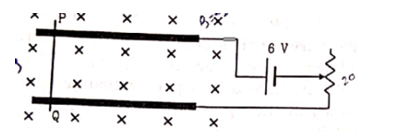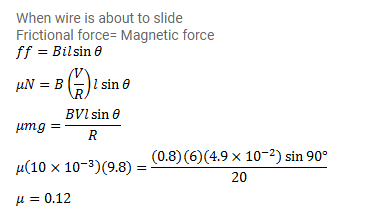# A metal wire PQ of mass 10g lies at rest on two horizontal metal rails separated by 4.90cm.

Question:

A metal wire $\mathrm{PQ}$ of mass $10 \mathrm{~g}$ lies at rest on two horizontal metal rails separated by $4.90 \mathrm{~cm}$. A vertically downward magnetic field of magnitude $0.800 \mathrm{~T}$ exists in the space. The resistance of the circuit is slowly decreased and it is found that when the resistance goes below $20.0 \Omega$, the wire $\mathrm{PQ}$ starts sliding on the rails. Find the coefficient of friction.Solution: